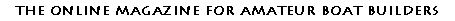NEW HOME INPUT INDEXES FEATURES CLASSIFIED CONTESTS ARCHIVES FORUM STORE LINKS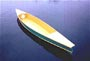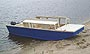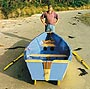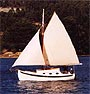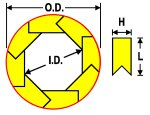Bird's Mouth Spars revisited
 Contents What is a bird's mouth spar? Some limits and possibilities explored Simple size calculations, the manual method Easiest size calculations, let your PC do the job! Dealing with a tapered mast Weight saving and strength loss Appendix

Introduction

A few months ago, an interesting article on bird's mouth spars was published right here, at Duckworks. The author, David Farless, presented a series of equations to accurately calculate the size of such a construction. This has inspired me to study this subject in greater depth. In this article, we will explore some of the limits and potentials of bird's mouth spars. You will also be provided with tools to let your computer do the size calculations for you. But first, a quick reminder...

What is a bird's mouth spar?

A bird's mouth spar is a way to make a hollow mast or spar from wood for use on a sailboat. Figure 1 below shows what the cross section looks like. It typically uses 8 identical pieces of wood or staves glued together. The outside is then trimmed to produce a round spar. The main goal is to save weight.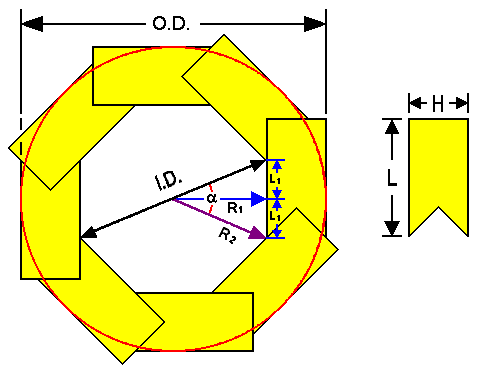Figure 1- Bird's Mouth Spar : Definitions

 O.D. is the outside diameter I.D. is the largest inside diameter R1 is the shortest inner radius R2 is the largest inner radius or half I.D. N is the number of sides (eight in this case)(alpha) is the angle between adjacent staves or 360° / N L is the width of material H is the thickness of material Important Ratios K is the thickness to width ratio of staves or (H / L) M is the "conversion factor" or (O.D. / L) A is the inside to outside diameter ratio or (I.D. / O.D.)

The definitions used here are pretty much the same that were used in the previous article. I have only added a few names for the ratios that will be of interest here. The model shown in Figure 1 is of "classic" proportions, that is, a stave's thickness ratio of 0.5 in an 8-sided configuration. Although I have never seen other configurations, it is possible to build a bird's mouth spar with a different number of sides. Figure 2 shows some of the possibilities.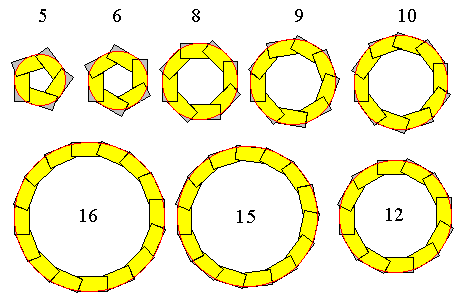Figure 2- The world of Bird's Mouth Spars

Okay, you might think that the math for size calculations would be far too complex for such configurations, right? Well, no! The equations presented by David Farless in the previously mentioned article had a hidden potential. With just a minor modification, these equations can handle any possible configuration! From now on, we will refer to these as the Farless equations. (Always give credit when credit is due!) The revised equations can be found in the Appendix section.

The trick for building a spar with more or less than 8 sides is in the V-notch angles used on the staves. Figure 3 shows them in a little bit more detail than Figure 2. Table 1 gives you the angles used and a few other points to take in consideration.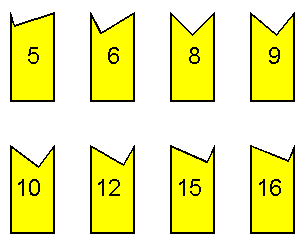Figure 3- Stave shape

 Table 1- Basic rules for bird's mouth spars Number of sides Angles for the V-notch (degrees) Theoretical limits for ratio(K) or (H /L) Practical range for ratio (K) N Angle 1 Angle 2 Minimum Maximum 5 72 18 0.1407 1.0515 not recommended 6 60 30 0.1200 1.1547 0.195 - 0.85 8 45 45 0.0929 1.4142 0.15 - 1 9 40 50 0.0834 1.5557 0.135 - 1.15 10 36 54 0.0757 1.7013 0.125 - 1.3 12 30 60 0.0637 2.0000 0.105 - 1.5 15 24 66 0.0515 2.4586 0.085 - 1.875 16 22.5 67.5 0.0483 2.6131 0.08 - 2 18 20 70 0.0431 2.9238 0.07 - 2.25 20 18 72 0.0389 3.2361 not recommended

Angle 1, shown in Figure 4 below, is located on the inside of the spar. Its value is always the same as "" (alpha), shown in Figure 1. Angle 2 is on the outside and is equal to 90 degrees minus the first angle.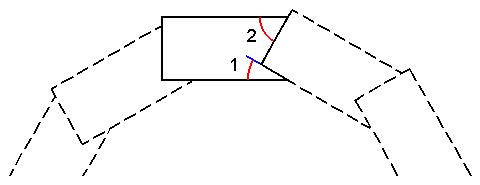Figure 4- Stave Angles

Table 1 also lists minimum and maximum limits for the thickness ratio of the staves (K). The minimum theoretical limit is reached when I.D. is equal to O.D. Figure 5 below shows an example of K going too far in that direction. In practice, you obviously need to use a higher ratio to have some thickness left! Otherwise your spar will fall apart when you try to round the outside. That's where the practical range comes into play. The minimum practical limits shown in Table 1 corresponds to the maximum weight saving possible for a given strength. (More on that later.)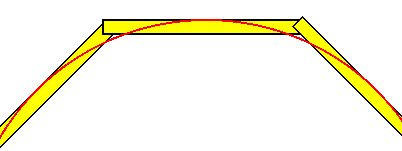Figure 5- Going too far: K is too low, I.D. is larger than O.D.!

The maximum theoretical limit is reached when I.D. equals zero (See Figure 6B). You can actually exceed that limit: the inside will open up again (Figure 6C). However, the formulas for calculating size can produce some weird results when you go in that zone. Better stay below that maximum limit. Here again, a practical upper limit for K has been shown. This maximum practical limit was chosen at the point where the weight saving falls to 5 percent compared to a solid spar of equal strength. (More on that later too.)Figure 6- Going too far, high values of K A- "Practical Maximum" B- "Theoretical Maximum", I.D. = 0 C- Gone too far, formula results unpredictable

You may have noticed that both the 5 and 20-sided configuration have the comment "not recommended". Here is why. For these, one of the angles used is very sharp. This means that the corresponding side of the V-notch becomes very thin (See Figure 3). This may be too fragile, at least during assembly. But this may very well be a weak spot under stress, even when glue has cured. This potential problem would only get worse for any larger number of sides and sets the limit of what you can do. This is one reason why 8-sided spars are far more common. For an 8-sided spar, the angles are equal and each side is of equal strength. And the glue area of the V-notch is also the largest at 45 degrees. So it is probably not a good idea to go very far from that 45-degree value. It may be wise to use a number of sides between 6 and 12. The smallest angle never falls below 30 degrees with these.

Configurations using 7, 11, 13, 14, 17 and 19 sides are not discussed here: the angles required with these are just too odd.

Now that we have looked at some of the limits of bird's mouth spars, let's look at ways to simplify size calculations.

Size Calculations

For those of you who have read the previous article written by David Farless, you already know that accurate size calculations are not really simple. That article also mentions a "rule-of-thumb" solution originating from a WoodenBoat article (I must admit that I have never read that article). The outside diameter was defined as 2.5 times the stave width. However that solution is not entirely accurate. In fact, both the width and thickness of the staves have an effect on the resulting diameter.

Wouldn't it be nice to have a solution that offers the simplicity of the WoodenBoat rule-of-thumb and the accuracy of the Farless equations at the same time? Better still, wouldn't it be great to have a computer program that does the calculations for you? Well, that is exactly what we'll do!

First, our new rule-of-thumb. If you look back at Figure 1, among the definitions, there is a ratio called "M". It is defined as O.D. divided by L. That is exactly the same thing as the "2.5x" from the WoodenBoat article. We "only" need to figure out what is the real value for "M". That ratio is defined in the Appendix if you are really "math curious". Table 2 below, however, will save you time. It gives you pre-calculated "M" values for 6 to 12-sided spars. The cells are highlighted in pink when K falls outside the "Practical Range" defined in Table 1. You should avoid these. No values are shown when K falls outside the theoretical limits of Table 1.

 Table 2- Improved "Rule-of-Thumb" : Conversion Factor (M) Stave Thickness Ratio (K) (M) 6 sides (M) 8 sides (M) 9 sides (M) 10 sides (M) 12 sides 0.100 - 2.444 2.771 3.097 3.745 0.125 1.795 2.451 2.777 3.102 3.749 0.150 1.807 2.458 2.783 3.106 3.752 0.175 1.820 2.465 2.788 3.111 3.755 0.200 1.832 2.473 2.794 3.116 3.759 0.225 1.845 2.480 2.800 3.121 3.762 0.250 1.857 2.487 2.806 3.125 3.766 0.275 1.870 2.495 2.812 3.130 3.769 0.300 1.882 2.502 2.818 3.135 3.772 0.325 1.895 2.509 2.824 3.140 3.776 0.350 1.907 2.517 2.829 3.145 3.779 0.375 1.920 2.524 2.835 3.149 3.782 0.400 1.932 2.531 2.841 3.154 3.786 0.425 1.945 2.539 2.847 3.159 3.789 0.450 1.957 2.546 2.853 3.164 3.792 0.475 1.970 2.553 2.859 3.168 3.796 0.500 1.982 2.561 2.864 3.173 3.799 0.525 1.995 2.568 2.870 3.178 3.802 0.550 2.007 2.575 2.876 3.183 3.806 0.575 2.020 2.583 2.882 3.187 3.809 0.600 2.032 2.590 2.888 3.192 3.812 0.625 2.045 2.597 2.894 3.197 3.816 0.650 2.057 2.605 2.900 3.202 3.819 0.675 2.070 2.612 2.905 3.207 3.822 0.700 2.082 2.619 2.911 3.211 3.826 0.725 2.095 2.627 2.917 3.216 3.829 0.750 2.107 2.634 2.923 3.221 3.833 0.775 2.120 2.641 2.929 3.226 3.836 0.800 2.132 2.649 2.935 3.230 3.839 0.825 2.145 2.656 2.940 3.235 3.843 0.850 2.157 2.663 2.946 3.240 3.846 0.875 2.170 2.670 2.952 3.245 3.849 0.900 2.182 2.678 2.958 3.250 3.853 0.925 2.195 2.685 2.964 3.254 3.856 0.950 2.207 2.692 2.970 3.259 3.859 0.975 2.220 2.700 2.976 3.264 3.863 1.000 2.232 2.707 2.981 3.269 3.866 1.025 2.245 2.714 2.987 3.273 3.869 1.050 2.257 2.722 2.993 3.278 3.873 1.075 2.270 2.729 2.999 3.283 3.876 1.100 2.282 2.736 3.005 3.288 3.879 1.125 2.295 2.744 3.011 3.293 3.883 1.150 2.307 2.751 3.017 3.297 3.886 1.175 - 2.758 3.022 3.302 3.889 1.200 - 2.766 3.028 3.307 3.893 1.225 - 2.773 3.034 3.312 3.896 1.250 - 2.780 3.040 3.316 3.900 1.275 - 2.788 3.046 3.321 3.903 1.300 - 2.795 3.052 3.326 3.906 1.325 - 2.802 3.057 3.331 3.910 1.350 - 2.810 3.063 3.336 3.913 1.375 - 2.817 3.069 3.340 3.916 1.400 - 2.824 3.075 3.345 3.920 1.425 - - 3.081 3.350 3.923 1.450 - - 3.087 3.355 3.926 1.475 - - 3.093 3.359 3.930 1.500 - - 3.098 3.364 3.933

Choose the stave thickness ratio and the number of sides that you want to use, look up the corresponding value of "M" in the table, and you have everything you need to do the size calculations. Let's do an example.

 Example 1 Let's say we want to build a 3-inch diameter, 8-sided spar. If we choose a stave thickness ratio (K) of 0.5, the resulting value for M is 2.561 (highlighted in yellow in Table 2). The value for the stave width (L) is then: L = O.D. / M L = 3 inches / 2.561 = 1.171 inches Since we chose a stave thickness ratio of 0.5, the thickness of the stave (H) is (0.5 X 1.171) or about 0.586 inches.

You can download a text version of Table 2 and print it on a single page. (You may have to adjust the page margins and the font size to achieve that). With this table at your disposal, you can do the calculations by hand if you have to.

Let's now look at the easiest method: your PC doing the calculations for you!

Bird's Mouth Spar Size Calculators

Instructions

Choose the Calculator that suits your needs. Enter the values of your choice on the left, then click on the "Calculate!" button. Results will appear on the right. An error message will appear in the Status box if your inputs don't work out. You can clear all entries by clicking on the Reload or Refresh button of your Web browser. Or you can just enter new inputs and click on "Calculate!" again. Clicking on the pictogram in the middle will lead you back to Figure 1, in case you need a reminder for all those definitions. Dimensions must all be in the same units. Ex.: Inputs in inches give results in inches.

Hints to avoid error messages (in the Status box)

Input values must be numbers higher than zero (no negatives, no letters).
The number of sides must be 5 or more.
I.D. must be smaller than O.D. (obviously).
H or K must not be too small or too large (less obvious).
H must be smaller than the radius or half the diameter.

Calculator 1: Given N, calculate the two angles
used for the V-notch on the staves.
 Input N: (Number of sides)
 Results Angle 1: degrees Angle 2: degrees

Status :

Calculator 2: Given N, O.D. and I.D., calculate L, H and K
 Inputs N: O.D.: I.D.:
 Results L : H : A : K : M :

Status :

Calculator 3: Given N, L and H, calculate O.D., I.D. and K
 Inputs N : L : H :
 Results O.D.: I.D.: A : K : M :

Status :

Calculator 4: Given N, O.D. and H, calculate L, I.D. and K
 Inputs N : O.D.: H :
 Results L : I.D.: A : K : M :

Status :

Calculator 5: Given N, O.D. and K, calculate L, H and I.D.
 Inputs N : O.D.: K :
 Results L : H : I.D.: A : M :

Status :

Calculator 6: Given N, L and K, calculate O.D., H and I.D.
 Inputs N : L : K :
 Results O.D.: H : I.D.: A : M :

Status :

Dealing with a Tapered Mast

There is one case where the Calculators above will prove handy: building a mast with a taper. A taper means that the mast diameter will gradually be reduced in size as you go up. I have never built a bird's mouth mast so far; I have only drafted a few in TurboCAD. So, I think the easiest way to build a tapered bird's mouth mast is to cut a taper in the width of the staves, leaving the thickness constant all the way. Figure 7 below illustrates this. As you can guess, the length has been condensed to better show what is going on.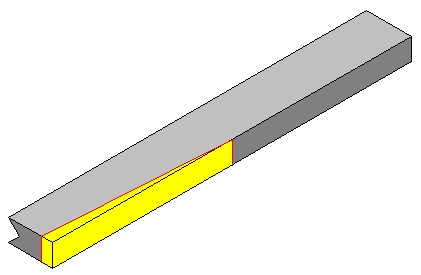Figure 7- Creating a taper : just cut away the yellow section

The yellow section would be removed to produce a tapered mast. This is the easiest cut to do: your piece of wood would be lying flat on its largest side. It sure beats cutting a taper on the V-notch side. It is also the best compromise for strength. By cutting this way, the thickness ratio would go up, minimizing the strength loss caused by a smaller end diameter. It also keeps the glue joint surface at its maximum.

Let's do an example. You can try it for yourself as we go along.

 Example 2 Step 1 Let's say we want to build a 3-inch diameter mast with a tapered top diameter of 2 inches. We will do a 12-sided mast this time. We can start with the bottom section of the mast using either Calculator 2, 4 or 5. Let's say we want to use O.D. and I.D. as inputs. This means we must choose Calculator 2 to do the job. Let's choose an I.D. of 1.75 inches. The results obtained for L and H are: L = 0.780 inch at the bottom H = 0.655 inch Step 2 Now, for the final dimensions at the top, we use Calculator 4 and enter our final O.D. of 2 inches and the value for H we have just calculated. The final stave width (L) we obtain for the top is: L = 0.512 inch at the top So we have to remove (0.780 - 0. 512) or 0.268 inch at the top, gradually reducing to zero at the point where we go back to the full 3-inch diameter.

This approach could be extended to multiple levels of taper.

Weight Saving and Strength Loss

We now arrive at the core of the subject: the weight saving that a bird's mouth spar can achieve. The information presented here is based in part on the "Farless equations", with one refinement. The formulas for relative mass and strength presented in that article were (and still are) first approximations. In the case of the strength formula, that approximation is also the worst-case figure, so it is a good idea to keep it as is.

The relative mass equation, though, can't be used to compare relative mass (or weight saving) for various configurations. It does not take into account the number of sides. Figure 8 below shows you why we must take that in account. It shows, using the same I.D. and O.D., a 6- and a 12-sided spar, superposed. The 12-sided spar area is shown in yellow; the 6-sided spar has additional area (in grey). Although these two examples have the same theoretical strength, the 6-sided spar is heavier.

The accurate formula for weight saving is substantially longer than the first approximation (see the Appendix Section).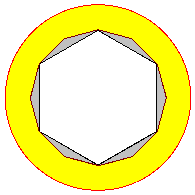Figure 8- Cross section area comparison, 6- and 12-sided configuration

So how does a bird's mouth spar compare to a solid spar in terms of weight saving and strength? To avoid comparing apples and oranges, we will use the ratio A or (I.D. / O.D.). First, Figure 9 below compares bird's mouth spars to a solid round spar of the same size or diameter. We can see that the weight saving increases with the number of sides. The difference is more important for high values of ratio A (thinner spar). The weight saving can look fantastic there, if you forget to look at the strength loss curve (in grey), which is the same for all configurations. You obviously don't get something for nothing. As ratio A comes closer to unity, you start to loose strength faster than you loose weight. Not an improvement. The range used for ratio A in these graphs stops at 0.2: at that point, the weight saving falls to about 5%, our "practical limit" for maximum K (See Table 1).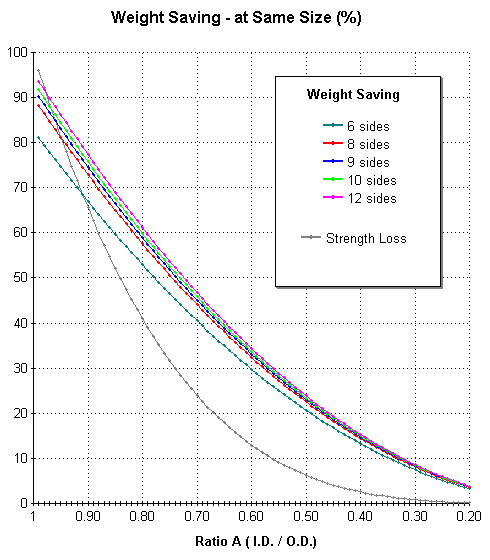Figure 9- Weight saving compared to a solid round spar of same size ...Round Exterior

There are two solutions to get a big weight saving without losing too much strength. First, use a larger diameter than the original solid spar. You can achieve the same theoretical strength and still save weight. Sounds too good to be true? There is one drawback: you end up with a larger spar. The larger the weight saving, the larger that spar becomes. You might end up with a spar that is just too large to be practical. Figure 10 below shows you what you can expect to achieve. The grey curve shows you how much bigger the spar must be to achieve the corresponding weight saving. We can see, for example, that a 12-sided spar can achieve a maximum weight saving of 70%! But to do so, its diameter must be about 90% larger than a solid spar! A less extreme example, the "classic" configuration (8-sided, K=0.5), requires a size only about 5% larger for equivalent strength, while saving about one-third in weight. Not bad!

(A note, here, you can use the Size Calculator #2 to figure what is the value of K for a given ratio A. Enter the number of sides you want to use, enter "1" for O.D. and enter the value A in the "I.D." input box. There is also a conversion table in the Appendix section.)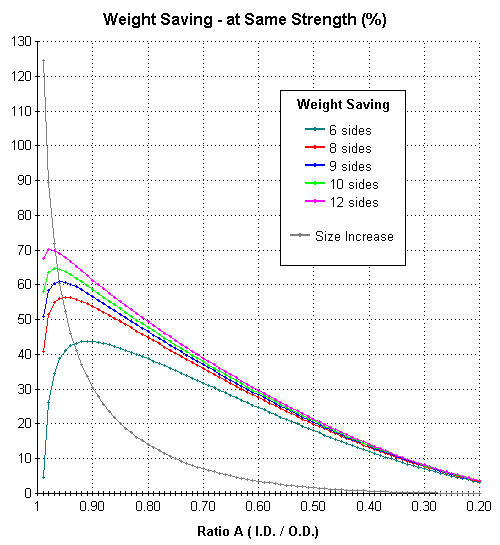Figure 10- Weight saving compared to a solid round spar of same strength ...Round Exterior

This graph reveals something interesting. As you go for a thinner spar, the weight saving goes up, until it reaches a peak. That peak, by the way, is the practical minimum ratio K proposed in Table 1. If you try a thinner value past that point, the weight saving starts to fall down again. This happens more or less in the graph area located on the left side of the "Size Increase" curve. You should avoid using values in that area: not only you won't save more weight, but also the size increase becomes enormous! There are two explanations for this phenomenon. This problem is due to the fact that the inside and outside shape are different. If both the inside and outside were the same shape, the weight saving would continue to climb on that graph. But because of the "corners" or vertex of the polygon shape, you end up with a big difference between minimum and maximum thickness. Those vertex are weak spots that put a limit on optimum weight saving. Another way to look at it is in the amount of material that you must remove to obtain a round exterior (See Figure 11). As a 12-sided spar is much "rounder" than a 6-sided, there is less waste. But even with a large number of sides, for very thin stave construction, you waste more material, weakening your spar.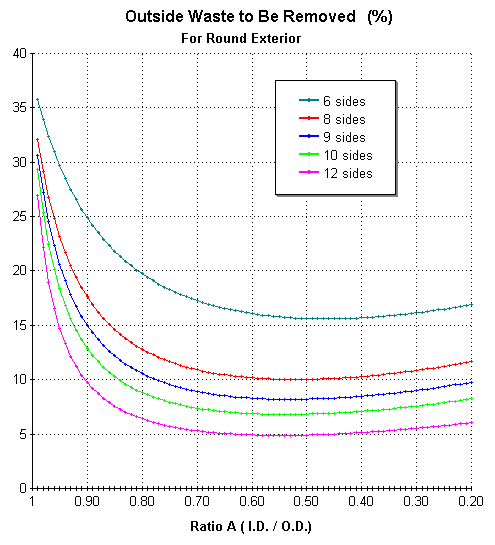Figure 11- Outside waste that must be removed to obtain a round exterior

The graph of Figure 10 tells you how to achieve the best weight saving, for a given strength. The highest results are achieved with a pretty large increase in size, perhaps too large. In real life, you would probably want a good compromise between weight, strength and size. Figure 12 below is an attempt at finding such a compromise. This Arbitrary Score is not a "scientific" calculation. The curves shown were obtained by subtracting the Size Increase curve from the Weight Saving curves of Figure 10. This is a "two-thumbs up" kind of rating. It rewards good weight saving but penalizes large size increases. It does shows where the overall good compromises are. You have to take the results with a grain of salt. You can get equal scores for very different weight saving values. On the left portion of the graph, high score values reflects great weight saving. On the right portion of the graph, high score values reflects minimal size increase. If weight saving is more important to you, go "left". If you want to replace an existing solid mast and you are limited in space, go "right".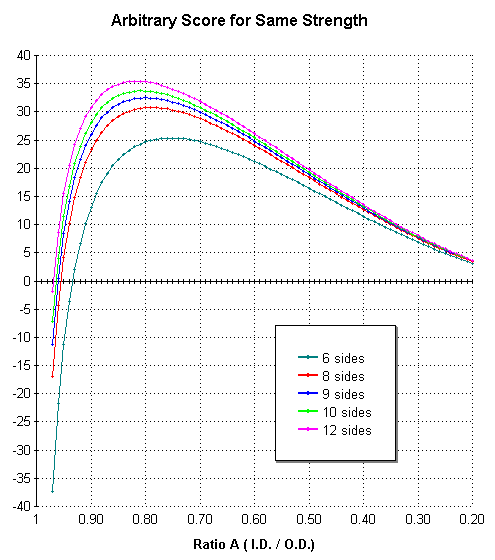Figure 12- Arbitrary Score compared to a solid round spar of same strength ...Round Exterior

The second method to maximize strength is to keep the exterior in the same polygon shape as the inside (See Figure 13). This is not as pretty, but you don't hit the same weight saving limit as a rounded bird's mouth spar. That's because the ratio A (I.D./O.D) is constant all around. And for a beginner like me, it might be easier to build. A 12-sided spar done this way wouldn't look too bad. For weight saving and strength comparison, we will use the minimum diameter for that polygon. This will allow us to use the same overall size calculations as a rounded spar.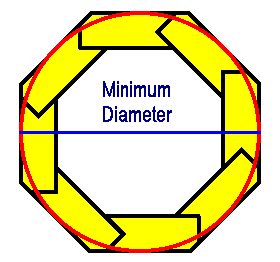Figure 13- Polygon exterior shape

Figure 14 compares a polygon-shaped bird's mouth spar to a solid round spar of equivalent strength. You can see, if you compare with Figure 10, that the weight saving can reach higher values. And the increase in size required to maintain strength is smaller. That's for high values of A. Although the graph stops at a maximum of one, ratio A can actually exceed one with such a configuration. That's because I.D. has been defined in diagonal, while O.D. is measured straight across.Figure 14- Weight saving compared to a solid round spar of same strength ...Polygon Exterior

Table 3 below gives you the maximum limit for (A). At that point, the stave thickness has reached zero.

 Table 3- Maximum theoretical values for ratio (A) - Polygon Exterior Number of sides (N) Maximum Ratio (A) (I.D. / O.D.) 5 1.2361 6 1.1547 8 1.0824 9 1.0642 10 1.0515 12 1.0353 15 1.0223 16 1.0196 18 1.0154 20 1.0124

When ratio (A) and the hollow area becomes small, you can reach a point where you no longer save weight! You end up with a higher weight than a solid round spar. So, for a thick stave, this configuration is not a good idea; the rounded version is a better choice for that.

Figure 15 below shows the Arbitrary Score for a polygon shape. The peak score is a bit higher than for a rounded version. But you hit negative values at the right end of the graph, just like Figure 14.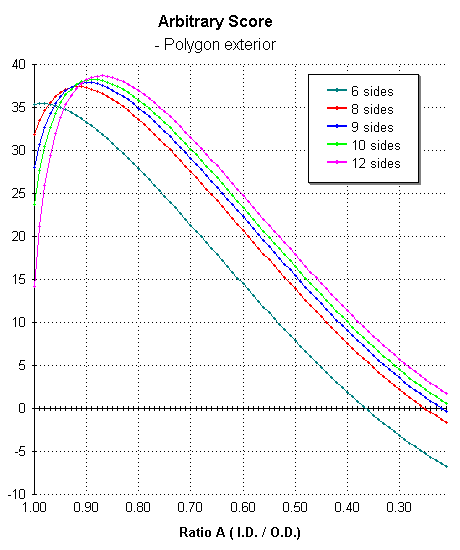Figure 15- Arbitrary Score compared to a solid round spar of same strength ...Polygon Exterior

The outside waste, for a polygon exterior, is obviously much smaller than for a round exterior, as can be seen in Figure 16 below. The waste increases as the hollow area becomes smaller.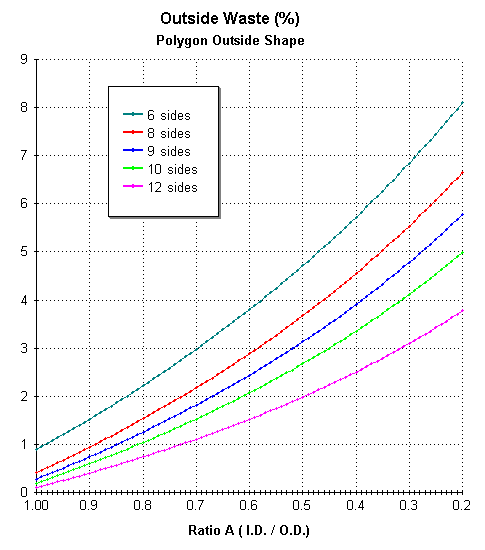Figure 16- Outside waste for a polygon exterior shape

You may have noticed, throughout these graphs, a large gap between the 6-sided and 8-sided configuration. That's because no 7-sided curves were done. This gap also tells you that an 8-sided spar would be a much better choice than a 6-sided one.

All the strength calculations are theoretical strength values. The formulas use what is called the moment of inertia. This is a fair estimate as long as the stress is uniformly distributed. In real life, there are always some localized stress points, like at the mast partner, for example. So you may have to add some extra reinforcements in critical areas to avoid bad surprises.

This about covers what I had to say about bird's mouth spars. I hope you have found this "exploration" interesting. Feel free to have a look at the Appendix section where the formulas used and the graph results in Table format are shown.

- The End -